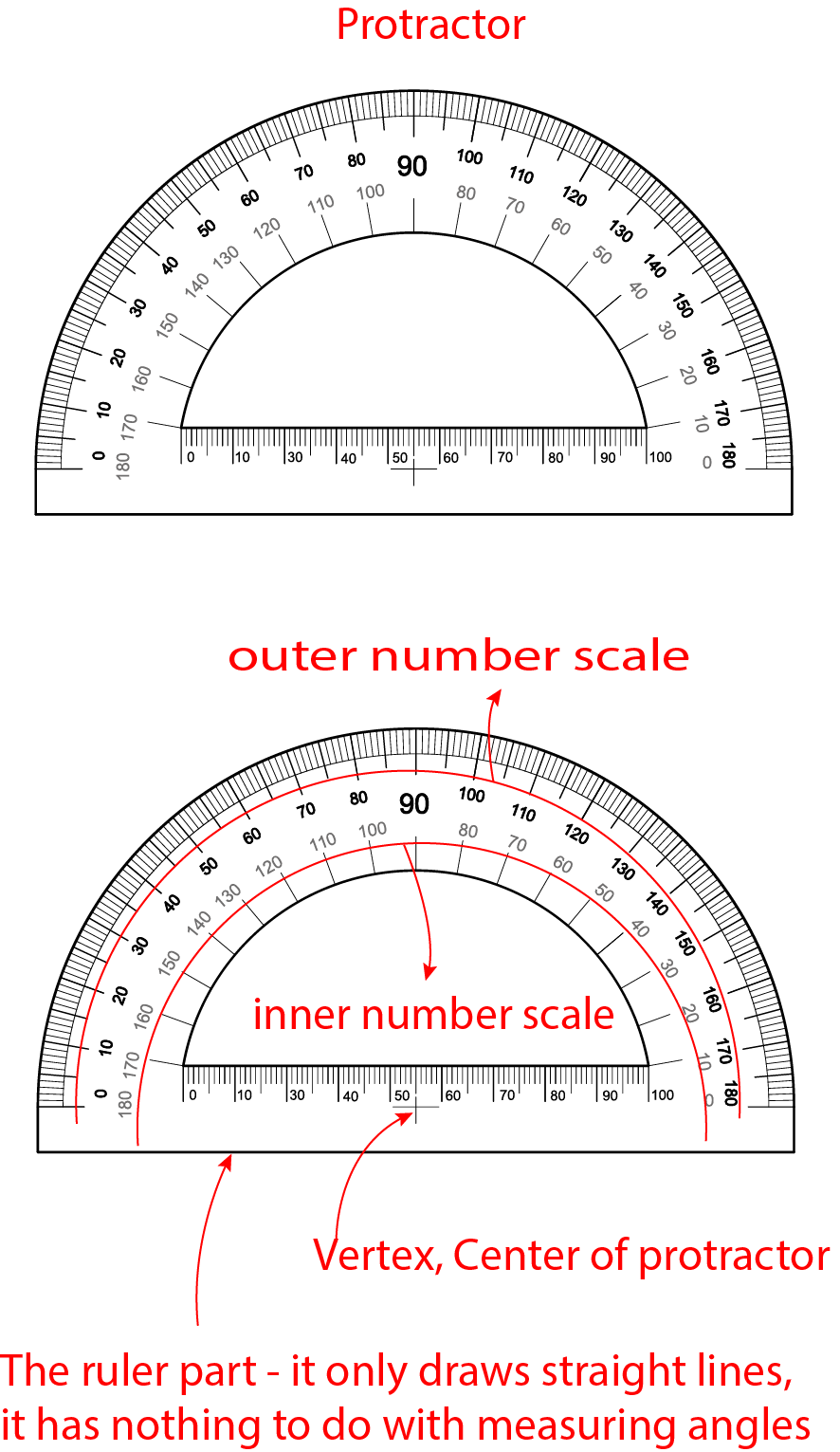# Measuring Angles Using A Protractor

Before we discuss how to measure an angle, let's get to know the protractor.

#Ruler part :

The ruler section is not used for measuring angles; it's solely for drawing straight lines. For instance, when we want to draw an angle of a specific degree, we use it to draw the arms of the angle or when drawing other polygons, etc. It contains the center of the angle.

Vertex or Center of protractor:
The most crucial part of the angle is its center (the pivot point). All angles are arranged around this center. If you pay attention, you'll see that the numbers face the center.

Outer and inner Number Scale
The part that measures the angle has degrees in opposite directions on the inside and outside. You'll see degrees going from right to left or from left to right. These allow us to measure from both arms of the angle. It can be used in a bidirectional manner. You'll see how in a moment.

# How to measure reflex angle ?

A reflex angle is an angle that measures more than 180 degree but less than 360 degree. Measuring a reflex angle directly can be a bit tricky with a standard protractor since most protractors only measure up to 180 degree. However, you can measure a reflex angle indirectly.

## Here's how:

Place the midpoint of the protractor at the vertex of the angle.
Measure the smaller angle (this is the acute or obtuse angle that is not the reflex angle).
Subtract the measurement of the smaller angle from 360°.

# Why Do We Measure Angles in 360 Degrees?

The measurement of angles in degrees is based on historical and practical reasons. Here are some insights on this topic.

Historical Origins: The ancient Babylonian civilization divided the year into 360 days. This was based on their observational astronomical data to estimate the duration of a year. The number 360 became a foundation for circular motions and cycles. Therefore, defining a full rotation of a circle as 360 degrees has its roots in this historical context.

Mathematical Convenience: 360 is a number divisible by many prime factors (e.g., 1, 2, 3, 4, 5, 6, 8, 9, 10, 12, 15, 18, 20, 24, 30, 36, 40, 45, 60, 72, 90, 120, 180, 360). This makes angle measurements and calculations related to circles easier.

Astronomical Observations: Ancient civilizations tried to measure time by observing the movements of stars and other celestial bodies in the sky. These observations contributed to the development of concepts related to circular motions and cycles.

Standardization: Over time, the 360-degree system became an international standard, ensuring that angle measurements in science, engineering, and daily life are consistent and understandable.

For these reasons, a full rotation is accepted as 360 degrees, and this has become a universal standard for measuring angles.